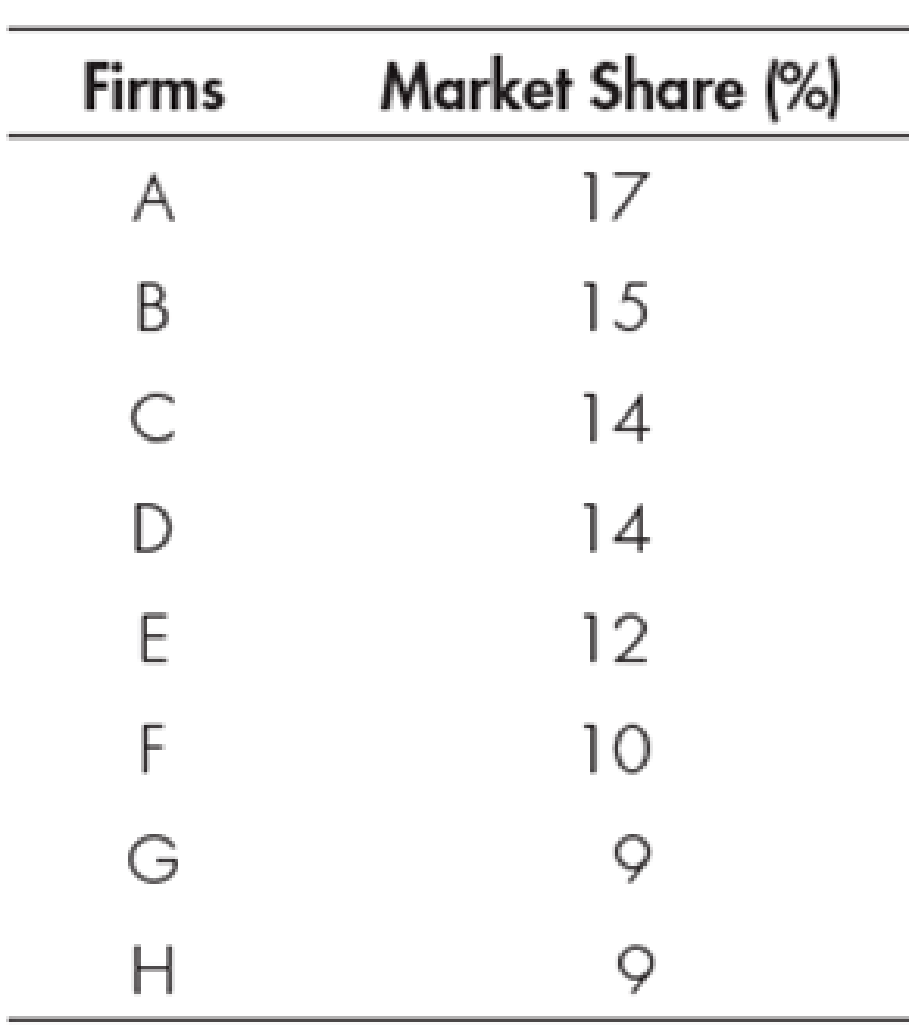Chapter 25, Problem 1WNG

Chapter
Section
Textbook Problem

Calculate the Herfindahl index and the four-firm concentration ratio for the following industry:To determine

Determine Herfindahl index and four-firm concentration ratio.

Explanation

The four-firm concentration ration can be calculated using the formula as follows:

Four-firm concentration ratio=Highest market share of the four firms        (1)

Herfindahl index can be calculated using the formula as follows:

Herfindahl index=(S1)2+(S2)2++(Sn)2        (2)

Here

S1 and Sn are the market shares.

Table-1 shows the value of the market share of the firms as follows:

Use Equation -1 to calculate the four-firm concentration ratio as follows:

Four-firm concentration ratio=17%+15%+14%+14%=60%

Therefore, the four-firm concentration ratio is 60%

Still sussing out bartleby?

Check out a sample textbook solution.

See a sample solution

The Solution to Your Study Problems

Bartleby provides explanations to thousands of textbook problems written by our experts, many with advanced degrees!

Get Started

Why do economists make assumptions?

Principles of Microeconomics (MindTap Course List)

TIME VALUE OF MONEY Answer the following questions: a. Assuming a rate of 10% annually, find the FV of 1,000 af...

Fundamentals of Financial Management, Concise Edition (with Thomson ONE - Business School Edition, 1 term (6 months) Printed Access Card) (MindTap Course List)

What is employee fraud?

Accounting Information Systems

Explain the matching principle.

College Accounting, Chapters 1-27 (New in Accounting from Heintz and Parry)

What is the AP/CD process?

Accounting Information Systems